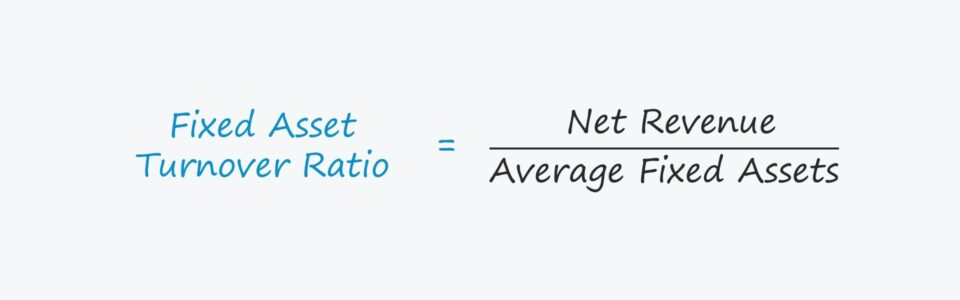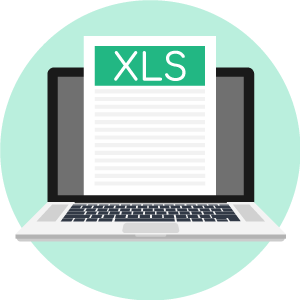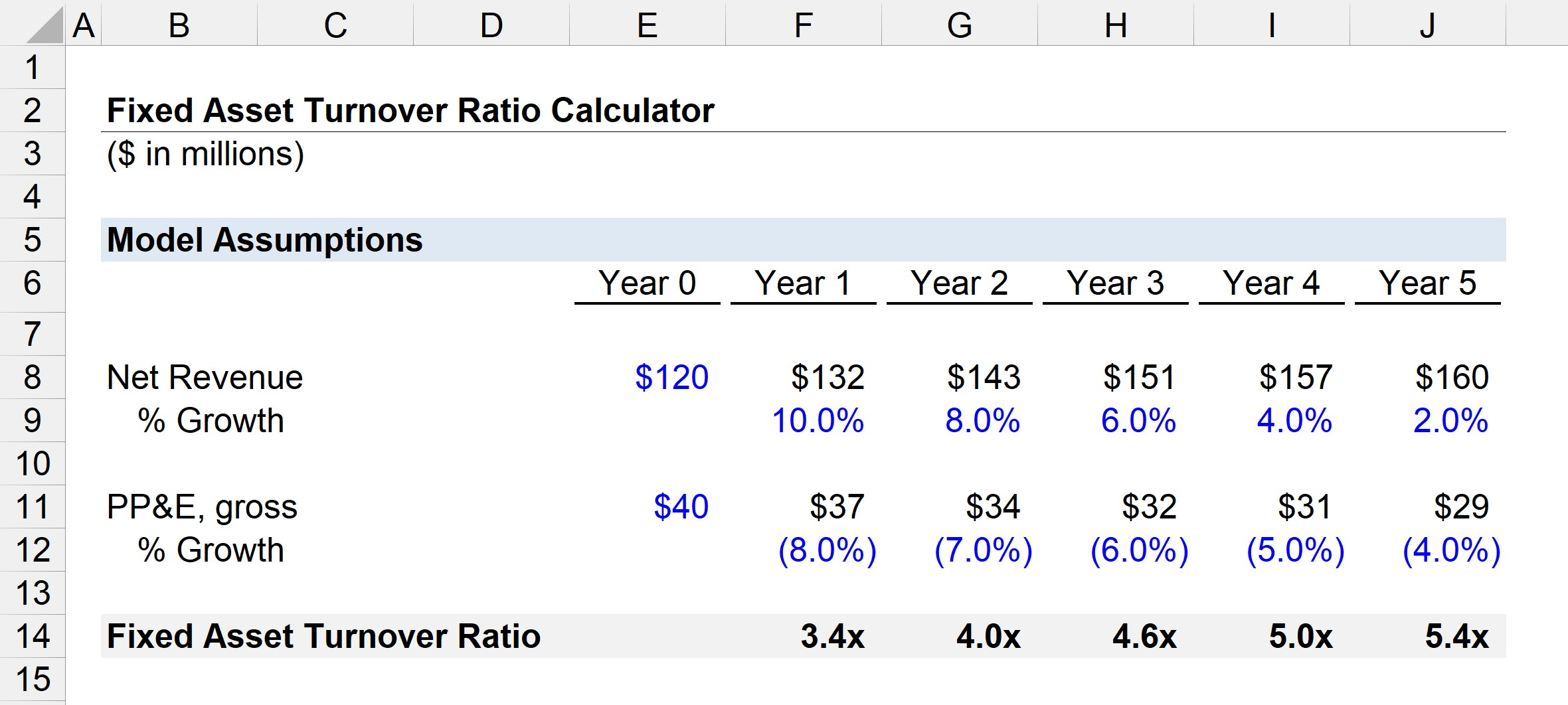# Fixed Asset Turnover Ratio

Understand the Fixed Asset Turnover Ratio• What does the fixed asset turnover ratio measure?
• What formula calculates the fixed asset turnover ratio?
• How is the fixed asset turnover different from the total asset turnover?
• Is a higher or lower fixed asset turnover ratio preferred?

## How to Calculate the Fixed Asset Turnover

The fixed asset turnover ratio, like the total asset turnover ratio, tracks how efficiently a company’s assets are being put to use (and producing sales).

However, the distinction is that the fixed asset turnover ratio formula includes solely long-term fixed assets, i.e. property, plant & equipment (PP&E), rather than all current and non-current assets.

Examples of fixed assets that provide long-term economic benefits (>1 year) include the following:

• Land and Property
• Buildings and Offices
• Machinery
• Equipment
• Vehicles

Therefore, the fixed asset turnover ratio determines if a company’s purchases of fixed assets – i.e. capital expenditures (CapEx) – are being spent effectively or not.

## Fixed Asset Turnover Ratio Formula

The formula to calculate the fixed asset turnover ratio compares a company’s net revenue to the average balance of fixed assets.

###### Fixed Asset Turnover Formula
• Fixed Asset Turnover Ratio = Net Revenue / Average Fixed Assets

## Fixed Asset Turnover – High or Low Ratio?

The fixed asset turnover ratio answers, “How much in revenue is generated per dollar of fixed asset owned?”

If a company’s fixed asset turnover is 2.0x, it is implied that each dollar of fixed assets owned results in \$2.00 of revenue.

In general, the higher the fixed asset turnover ratio, the better, as the company is implied to be generating more revenue per dollar of long-term assets owned.

• If a company’s fixed asset turnover is high, it is implied that the company is purchasing long-term assets efficiently.
• If a company’s fixed asset turnover is low, on the other hand, this indicates the company is NOT receiving sufficient value (i.e. revenue) in return from its long-term assets.

Given how costly fixed asset purchases can be – on the initial date of purchase as well as the associated maintenance (or replacement) expenses – CapEx decisions must be made carefully.

Otherwise, operating inefficiencies can be created that have significant implications (i.e. long-lasting consequences) and have the potential to erode a company’s profit margins.

## Fixed Asset Turnover Ratio by Industry

After calculating the fixed asset turnover ratio, the metric can be compared across historical periods to assess trends.

Comparisons to the ratios of industry peers can gauge how a company fares against its competitors regarding its spending on long-term assets (i.e. whether it is more efficient or lagging behind peers).

But in order to be useful, the ratio must be compared to industry comparables, or companies with similar characteristics as the target company, such as similar business models, end markets, and risks.

The average fixed asset turnover ratio varies across industries.

For instance, comparisons between capital-intensive (“asset-heavy”) industries cannot be made with “asset-lite” industries since their business models and reliance on long-term assets are too different.

In particular, CapEx spending patterns in recent periods must also be understood when making comparisons, since one-time periodic purchases could be misleading and skew the ratio.

## Fixed Asset Turnover Ratio Calculator – Excel Template

We’ll now move to a modeling exercise, which you can access by filling out the form below.Submitting ...

## Fixed Asset Turnover Ratio Example Calculation

Suppose an industrials company generated \$120 million in net revenue in the past year, with \$40 million in PP&E.

After that year, the company’s revenue grows by 10%, with the growth rate then stepping down by 2% per year.

The company’s PP&E, the only fixed asset on its balance sheet, falls by 8% after Year 0 – with the growth rate then stepping up by 1% each year in each subsequent period (i.e. to negative 4% by the end of the projection period).

• Year 0 → Year 1: 10% Revenue Growth; – 8% PP&E Growth
• Year 1 → Year 2: 8% Revenue Growth; – 7% PP&E Growth
• Year 2 → Year 3: 6% Revenue Growth; – 6% PP&E Growth
• Year 3 → Year 4: 4% Revenue Growth; – 5% PP&E Growth
• Year 4 → Year 5: 2% Revenue Growth; – 4% PP&E Growth

From Year 0 to the end of Year 5, the company’s net revenue expands from \$120 million to \$160 million, whereas its PP&E declines from \$40 million to \$29 million.

In our hypothetical scenario, we can assume that the company’s revenue model is shifting from being predominantly comprised of one-time expensive purchases to recurring component purchases and services related to maintenance.

Unlike the initial equipment sale, the revenue from recurring component purchases and services provided to existing customers requires less spending on long-term assets.

For example, inventory purchases or hiring technical staff to service customers is cheaper than major CapEx.

We can now calculate the fixed asset turnover ratio by dividing the net revenue for the year by the average fixed asset balance, which is equal to the sum of the current and prior period balance divided by two.

• Fixed Asset Turnover = Net Revenue / Average (Current, Prior Period Fixed Asset Balance)

The calculated fixed asset turnover ratios from Year 1 to Year 5 are as follows.

• Year 1 = 3.4x
• Year 2 = 4.0x
• Year 3 = 4.6x
• Year 4 = 5.0x
• Year 5 = 5.4x

Despite the reduction in CapEx, the company’s revenue is growing – higher revenue is being generated on lower levels of CapEx purchases.

Since the company’s revenue growth remains strong throughout the forecast period while its CapEx spending declined, the fixed asset turnover trends upward.Inline FeedbacksLearn Financial Modeling Online

Everything you need to master financial and valuation modeling: 3-Statement Modeling, DCF, Comps, M&A and LBO.

X

The Wall Street Prep Quicklesson Series

7 Free Financial Modeling Lessons

Get instant access to video lessons taught by experienced investment bankers. Learn financial statement modeling, DCF, M&A, LBO, Comps and Excel shortcuts.